# Binary Heaps Notes | Study GATE Computer Science Engineering(CSE) 2023 Mock Test Series - GATE

## GATE: Binary Heaps Notes | Study GATE Computer Science Engineering(CSE) 2023 Mock Test Series - GATE

The document Binary Heaps Notes | Study GATE Computer Science Engineering(CSE) 2023 Mock Test Series - GATE is a part of the GATE Course GATE Computer Science Engineering(CSE) 2023 Mock Test Series.
All you need of GATE at this link: GATE

## Binary Heap

A Binary Heap is a Binary Tree with following properties.
1) It’s a complete tree (All levels are completely filled except possibly the last level and the last level has all keys as left as possible). This property of Binary Heap makes them suitable to be stored in an array.

2) A Binary Heap is either Min Heap or Max Heap. In a Min Binary Heap, the key at root must be minimum among all keys present in Binary Heap. The same property must be recursively true for all nodes in Binary Tree. Max Binary Heap is similar to Min Heap.

Examples of Min Heap: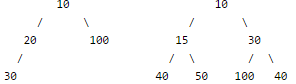How is Binary Heap represented?

A Binary Heap is a Complete Binary Tree. A binary heap is typically represented as array. Please refer below article for details.
Array Representation of Binary Heap

Applications of Heaps:
1) Heap Sort: Heap Sort uses Binary Heap to sort an array in O(nLogn) time.

2) Priority Queue: Priority queues can be efficiently implemented using Binary Heap because it supports insert(), delete() and extractmax(), decreaseKey() operations in O(logn) time. Binomoial Heap and Fibonacci Heap are variations of Binary Heap. These variations perform union also efficiently.

3) Graph Algorithms: The priority queues are especially used in Graph Algorithms like Dijkstra`s Shortest Path andPrim`s minimum Spanning Tree.

Operations on Min Heap:
1) getMini(): It returns the root element of Min Heap. Time Complexity of this operation is O(1).

2) extractMin(): Removes the minimum element from Min Heap. Time Complexity of this Operation is O(Logn) as this operation needs to maintain the heap property (by calling heapify()) after removing root.

3) decreaseKey(): Decreases value of key. Time complexity of this operation is O(Logn). If the decreases key value of a node is greater than parent of the node, then we don’t need to do anything. Otherwise, we need to traverse up to fix the violated heap property.

4) insert(): Inserting a new key takes O(Logn) time. We add a new key at the end of the tree. IF new key is greater than its parent, then we don’t need to do anything. Otherwise, we need to traverse up to fix the violated heap property.

5) delete(): Deleting a key also takes O(Logn) time. We replace the key to be deleted with minum infinite by calling decreaseKey(). After decreaseKey(), the minus infinite value must reach root, so we call extractMin() to remove key.

Following is C++ implementation of basic heap operations.

// A C++ program to demonstrate common Binary Heap Operations
#include<iostream>
#include<climits>
using namespace std;

// Prototype of a utility function to swap two integers
void swap(int *x, int *y);

// A class for Min Heap
class MinHeap
{
int *harr; // pointer to array of elements in heap
int capacity; // maximum possible size of min heap
int heap_size; // Current number of elements in min heap
public:
// Constructor
MinHeap(int capacity);

// to heapify a subtree with root at given index
void MinHeapify(int );

int parent(int i) { return (i-1)/2; }

// to get index of left child of node at index i
int left(int i) { return (2*i + 1); }

// to get index of right child of node at index i
int right(int i) { return (2*i + 2); }

// to extract the root which is the minimum element
int extractMin();

// Decreases key value of key at index i to new_val
void decreaseKey(int i, int new_val);

// Returns the minimum key (key at root) from min heap
int getMin() { return harr; }

// Deletes a key stored at index i
void deleteKey(int i);

// Inserts a new key 'k'
void insertKey(int k);
};

// Constructor: Builds a heap from a given array a[] of given size
MinHeap::MinHeap(int cap)
{
heap_size = 0;
capacity = cap;
harr = new int[cap];
}

// Inserts a new key 'k'
void MinHeap::insertKey(int k)
{
if (heap_size == capacity)
{
cout << " Overflow: Could not insertKey ";
return;
}

// First insert the new key at the end
heap_size++;
int i = heap_size - 1;
harr[i] = k;

// Fix the min heap property if it is violated
while (i != 0 && harr[parent(i)] > harr[i])
{
swap(&harr[i], &harr[parent(i)]);
i = parent(i);
}
}

// Decreases value of key at index 'i' to new_val.  It is assumed that
// new_val is smaller than harr[i].
void MinHeap::decreaseKey(int i, int new_val)
{
harr[i] = new_val;
while (i != 0 && harr[parent(i)] > harr[i])
{
swap(&harr[i], &harr[parent(i)]);
i = parent(i);
}
}

// Method to remove minimum element (or root) from min heap
int MinHeap::extractMin()
{
if (heap_size <= 0)
return INT_MAX;
if (heap_size == 1)
{
heap_size--;
return harr;
}

// Store the minimum value, and remove it from heap
int root = harr;
harr = harr[heap_size-1];
heap_size--;
MinHeapify(0);

return root;
}

// This function deletes key at index i. It first reduced value to minus
// infinite, then calls extractMin()
void MinHeap::deleteKey(int i)
{
decreaseKey(i, INT_MIN);
extractMin();
}

// A recursive method to heapify a subtree with root at given index
// This method assumes that the subtrees are already heapified
void MinHeap::MinHeapify(int i)
{
int l = left(i);
int r = right(i);
int smallest = i;
if (l < heap_size && harr[l] < harr[i])
smallest = l;
if (r < heap_size && harr[r] < harr[smallest])
smallest = r;
if (smallest != i)
{
swap(&harr[i], &harr[smallest]);
MinHeapify(smallest);
}
}

// A utility function to swap two elements
void swap(int *x, int *y)
{
int temp = *x;
*x = *y;
*y = temp;
}

// Driver program to test above functions
int main()
{
MinHeap h(11);
h.insertKey(3);
h.insertKey(2);
h.deleteKey(1);
h.insertKey(15);
h.insertKey(5);
h.insertKey(4);
h.insertKey(45);
cout << h.extractMin() << " ";
cout << h.getMin() << " ";
h.decreaseKey(2, 1);
cout << h.getMin();
return 0;
}

## Time Complexity of building a heap

Consider the following algorithm for building a Heap of an input array A.

BUILD-HEAP(A)
heapsize := size(A);
for i := floor(heapsize/2) downto 1
do HEAPIFY(A, i);
end for
END

What is the worst case time complexity of the above algo?
Although the worst case complexity looks like O(nLogn), upper bound of time complexity is O(n).

## Applications of Heap Data Structure

Heap Data Structure is generally taught with Heapsort. Heapsort algorithm has limited uses because Quicksort is better in practice. Nevertheless, the Heap data structure itself is enormously used. Following are some uses other than Heapsort.

Priority Queues: Priority queues can be efficiently implemented using Binary Heap because it supports insert(), delete() and extractmax(), decreaseKey() operations in O(logn) time. Binomoial Heap and Fibonacci Heap are variations of Binary Heap. These variations perform union also in O(logn) time which is a O(n) operation in Binary Heap. Heap Implemented priority queues are used in Graph algorithms like Prim`s Algorithm and Dijkstra`s algorithm.

Order statistics: The Heap data structure can be used to efficiently find the kth smallest (or largest) element in an array.

Why is Binary Heap Preferred over BST for Priority Queue?

A typical Priority Queue requires following operations to be efficient.

1. Get Top Priority Element (Get minimum or maximum)
2. Insert an element
3. Remove top priority element
4. Decrease Key

A Binary Heap supports above operations with following time complexities:

1. O(1)
2. O(Logn)
3. O(Logn)
4. O(Logn)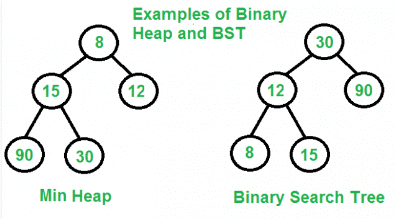A Self Balancing Binary Search Tree like  AVL Tree, Red-Black Tree, etc can also support above operations with same time complexities.

1. Finding minimum and maximum are not naturally O(1), but can be easily implemented in O(1) by keeping an extra pointer to minimum or maximum and updating the pointer with insertion and deletion if required. With deletion we can update by finding inorder predecessor or successor.
2. Inserting an element is naturally O(Logn)
3. Removing maximum or minimum are also O(Logn)
4. Decrease key can be done in O(Logn) by doing a deletion followed by insertion.

So why is Binary Heap Preferred for Priority Queue?

• Since Binary Heap is implemented using arrays, there is always better locality of reference and operations are more cache friendly.
• Although operations are of same time complexity, constants in Binary Search Tree are higher.
• We can build a Binary Heap in O(n) time. Self Balancing BSTs require O(nLogn) time to construct.
• Binary Heap doesn’t require extra space for pointers.
• Binary Heap is easier to implement.
• There are variations of Binary Heap like Fibonacci Heap that can support insert and decrease-key in Θ(1) time

Is Binary Heap always better?

Although Binary Heap is for Priority Queue, BSTs have their own advantages and the list of advantages is in-fact bigger compared to binary heap.

• Searching an element in self-balancing BST is O(Logn) which is O(n) in Binary Heap.
• We can print all elements of BST in sorted order in O(n) time, but Binary Heap requires O(nLogn) time.
• Floor and ceil can be found in O(Logn) time.
• K`th largest/smallest element be found in O(Logn) time by augmenting tree with an additional field.

## Heap Sort

Heap sort is a comparison based sorting technique based on Binary Heap data structure. It is similar to selection sort where we first find the maximum element and place the maximum element at the end. We repeat the same process for remaining element.

What is Binary Heap?

Let us first define a Complete Binary Tree. A complete binary tree is a binary tree in which every level, except possibly the last, is completely filled, and all nodes are as far left as possible.

A  Binary Heap is a Complete Binary Tree where items are stored in a special order such that value in a parent node is greater(or smaller) than the values in its two children nodes. The former is called as max heap and the latter is called min heap. The heap can be represented by binary tree or array.

Why array based representation for Binary Heap?

Since a Binary Heap is a Complete Binary Tree, it can be easily represented as array and array based representation is space efficient. If the parent node is stored at index I, the left child can be calculated by 2 * I + 1 and right child by 2 * I + 2 (assuming the indexing starts at 0).

Heap Sort Algorithm for sorting in increasing order:
1. Build a max heap from the input data.
2. At this point, the largest item is stored at the root of the heap. Replace it with the last item of the heap followed by reducing the size of heap by 1. Finally, heapify the root of tree.
3. Repeat above steps while size of heap is greater than 1.

How to build the heap?

Heapify procedure can be applied to a node only if its children nodes are heapified. So the heapification must be performed in the bottom up order.

Lets understand with the help of an example: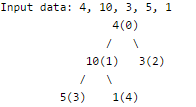The numbers in bracket represent the indices in the array
representation of data.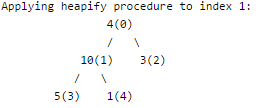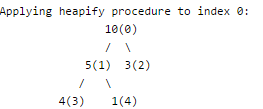The heapify procedure calls itself recursively to build heap
in top down manner.

C++JavaPython
// C++ program for implementation of Heap Sort
#include <iostream>
using namespace std;

// To heapify a subtree rooted with node i which is
// an index in arr[]. n is size of heap
void heapify(int arr[], int n, int i)
{
int largest = i;  // Initialize largest as root
int l = 2*i + 1;  // left = 2*i + 1
int r = 2*i + 2;  // right = 2*i + 2

// If left child is larger than root
if (l < n && arr[l] > arr[largest])
largest = l;

// If right child is larger than largest so far
if (r < n && arr[r] > arr[largest])
largest = r;

// If largest is not root
if (largest != i)
{
swap(arr[i], arr[largest]);

// Recursively heapify the affected sub-tree
heapify(arr, n, largest);
}
}

// main function to do heap sort
void heapSort(int arr[], int n)
{
// Build heap (rearrange array)
for (int i = n / 2 - 1; i >= 0; i--)
heapify(arr, n, i);

// One by one extract an element from heap
for (int i=n-1; i>=0; i--)
{
// Move current root to end
swap(arr, arr[i]);

// call max heapify on the reduced heap
heapify(arr, i, 0);
}
}

/* A utility function to print array of size n */
void printArray(int arr[], int n)
{
for (int i=0; i<n; ++i)
cout << arr[i] << " ";
cout << " ";
}

// Driver program
int main()
{
int arr[] = {12, 11, 13, 5, 6, 7};
int n = sizeof(arr)/sizeof(arr);

heapSort(arr, n);

cout << "Sorted array is ";
printArray(arr, n);
}

Output:
Sorted array is
5 6 7 11 12 13
Here is previous C code for reference.
Notes:
Heap sort is an in-place algorithm.
Its typical implementation is not stable, but can be made stable (See this)
Time Complexity: Time complexity of heapify is O(Logn). Time complexity of createAndBuildHeap() is O(n) and overall time complexity of Heap Sort is O(nLogn).

## Sort a nearly sorted (or K sorted) array

Given an array of n elements, where each element is at most k away from its target position, devise an algorithm that sorts in O(n log k) time.
For example, let us consider k is 2, an element at index 7 in the sorted array, can be at indexes 5, 6, 7, 8, 9 in the given array.

We can use Insertion Sort to sort the elements efficiently. Following is the C code for standard Insertion Sort.

/* Function to sort an array using insertion sort*/
void insertionSort(int A[], int size)
{
int i, key, j;
for (i = 1; i < size; i++)
{
key = A[i];
j = i-1;

/* Move elements of A[0..i-1], that are greater than key, to one
position ahead of their current position.
This loop will run at most k times */
while (j >= 0 && A[j] > key)
{
A[j+1] = A[j];
j = j-1;
}
A[j+1] = key;
}
}

The inner loop will run at most k times. To move every element to its correct place, at most k elements need to be moved. So overall complexity will be O(nk)

We can sort such arrays more efficiently with the help of Heap data structure. Following is the detailed process that uses Heap.
1) Create a Min Heap of size k+1 with first k+1 elements. This will take O(k) time.
2) One by one remove min element from heap, put it in result array, and add a new element to heap from remaining elements.

Removing an element and adding a new element to min heap will take Logk time. So overall complexity will be O(k) + O((n-k)*logK)

#include<iostream>
using namespace std;

// Prototype of a utility function to swap two integers
void swap(int *x, int *y);

// A class for Min Heap
class MinHeap
{
int *harr; // pointer to array of elements in heap
int heap_size; // size of min heap
public:
// Constructor
MinHeap(int a[], int size);

// to heapify a subtree with root at given index
void MinHeapify(int );

// to get index of left child of node at index i
int left(int i) { return (2*i + 1); }

// to get index of right child of node at index i
int right(int i) { return (2*i + 2); }

// to remove min (or root), add a new value x, and return old root
int replaceMin(int x);

// to extract the root which is the minimum element
int extractMin();
};

// Given an array of size n, where every element is k away from its target
// position, sorts the array in O(nLogk) time.
int sortK(int arr[], int n, int k)
{
// Create a Min Heap of first (k+1) elements from
// input array
int *harr = new int[k+1];
for (int i = 0; i<=k && i<n; i++) // i < n condition is needed when k > n
harr[i] = arr[i];
MinHeap hp(harr, k+1);

// i is index for remaining elements in arr[] and ti
// is target index of for cuurent minimum element in
// Min Heapm 'hp'.
for(int i = k+1, ti = 0; ti < n; i++, ti++)
{
// If there are remaining elements, then place
// root of heap at target index and add arr[i]
// to Min Heap
if (i < n)
arr[ti] = hp.replaceMin(arr[i]);

// Otherwise place root at its target index and
// reduce heap size
else
arr[ti] = hp.extractMin();
}
}

// FOLLOWING ARE IMPLEMENTATIONS OF STANDARD MIN HEAP METHODS FROM CORMEN BOOK
// Constructor: Builds a heap from a given array a[] of given size
MinHeap::MinHeap(int a[], int size)
{
heap_size = size;
harr = a;  // store address of array
int i = (heap_size - 1)/2;
while (i >= 0)
{
MinHeapify(i);
i--;
}
}

// Method to remove minimum element (or root) from min heap
int MinHeap::extractMin()
{
int root = harr;
if (heap_size > 1)
{
harr = harr[heap_size-1];
heap_size--;
MinHeapify(0);
}
return root;
}

// Method to change root with given value x, and return the old root
int MinHeap::replaceMin(int x)
{
int root = harr;
harr = x;
if (root < x)
MinHeapify(0);
return root;
}

// A recursive method to heapify a subtree with root at given index
// This method assumes that the subtrees are already heapified
void MinHeap::MinHeapify(int i)
{
int l = left(i);
int r = right(i);
int smallest = i;
if (l < heap_size && harr[l] < harr[i])
smallest = l;
if (r < heap_size && harr[r] < harr[smallest])
smallest = r;
if (smallest != i)
{
swap(&harr[i], &harr[smallest]);
MinHeapify(smallest);
}
}

// A utility function to swap two elements
void swap(int *x, int *y)
{
int temp = *x;
*x = *y;
*y = temp;
}

// A utility function to print array elements
void printArray(int arr[], int size)
{
for (int i=0; i < size; i++)
cout << arr[i] << " ";
cout << endl;
}

// Driver program to test above functions
int main()
{
int k = 3;
int arr[] = {2, 6, 3, 12, 56, 8};
int n = sizeof(arr)/sizeof(arr);
sortK(arr, n, k);

cout << "Following is sorted array ";
printArray (arr, n);

return 0;
}

Output:

Following is sorted array
2 3 6 8 12 56

The Min Heap based method takes O(nLogk) time and uses O(k) auxiliary space.

We can also use a Balanced Binary Search Tree instead of Heap to store K+1 elements. The insert and delete operations on Balanced BST also take O(Logk) time. So Balanced BST based method will also take O(nLogk) time, but the Heap bassed method seems to be more efficient as the minimum element will always be at root. Also, Heap doesn’t need extra space for left and right pointers.

The document Binary Heaps Notes | Study GATE Computer Science Engineering(CSE) 2023 Mock Test Series - GATE is a part of the GATE Course GATE Computer Science Engineering(CSE) 2023 Mock Test Series.
All you need of GATE at this link: GATEUse Code STAYHOME200 and get INR 200 additional OFF

## GATE Computer Science Engineering(CSE) 2023 Mock Test Series

136 docs|165 tests

Track your progress, build streaks, highlight & save important lessons and more!

,

,

,

,

,

,

,

,

,

,

,

,

,

,

,

,

,

,

,

,

,

;